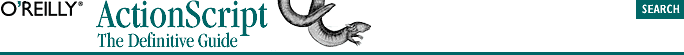home | O'Reilly's CD bookshelfs | FreeBSD | Linux | Cisco | Cisco Exam## 4.4. Working with Numbers

We can manipulate numbers by combining them with operators to form mathematical expressions, and by calling built-in functions to perform complex mathematical operations.

### 4.4.1. Using Operators

Basic arithmetic -- addition, subtraction, multiplication, and division -- is accomplished using the +, -, *, and / operators. Operators can be used with any numeric literals or data containers such as variables. Mathematical expressions are evaluated in the order determined by the precedence of the operators as shown in Table 5-1. For example, multiplication is performed before addition. All of these are legitimate uses of mathematical operators:

```x = 3 * 5;            // Assign the value 15 to x
x = 1 + 2 - 3 / 4;    // Assign the value 2.25 to x

x = 56;
y = 4 * 6 + x;        // Assign the value 80 to y
y = x + (x * x) / x;  // Assign the value 112 to y```